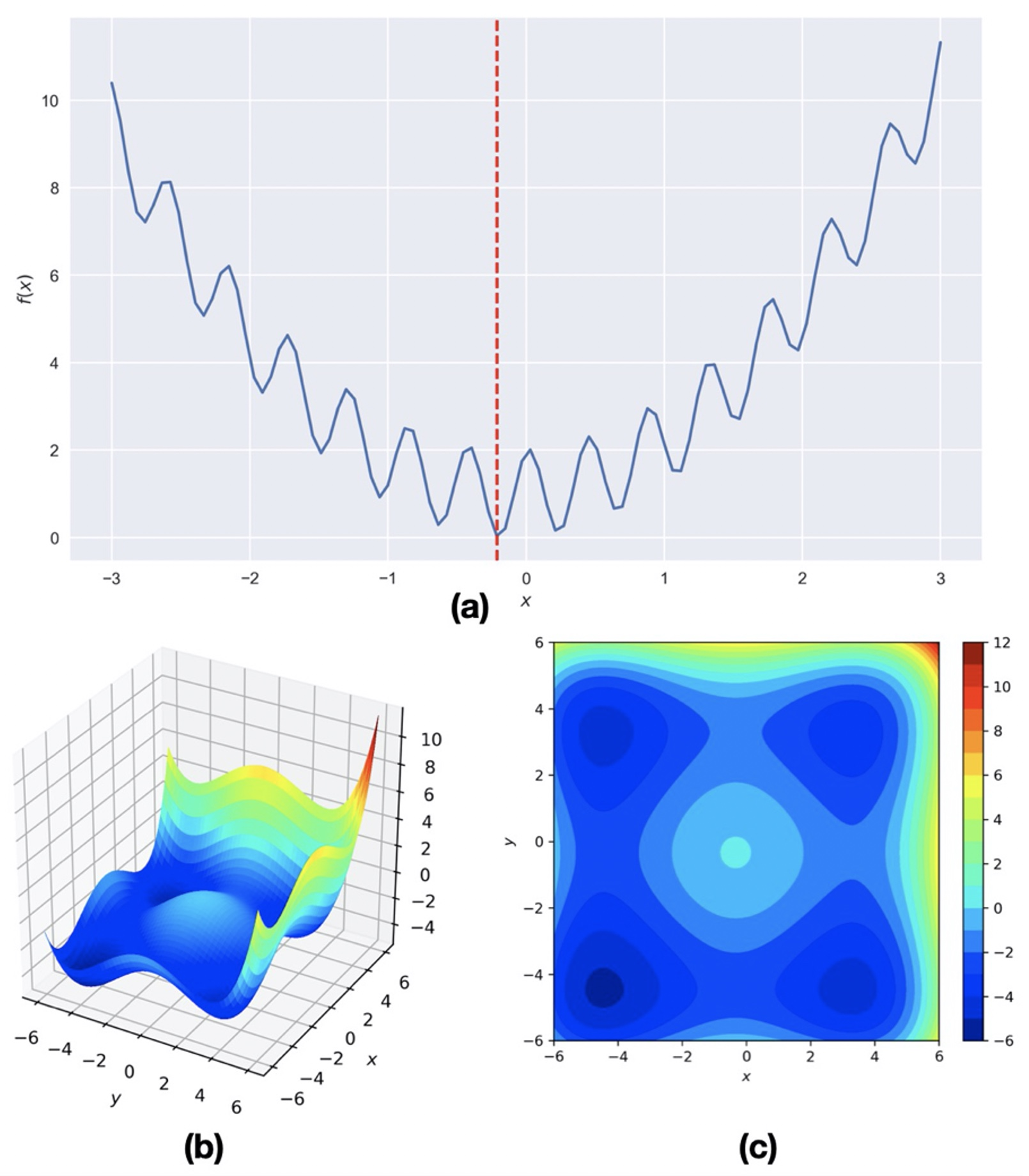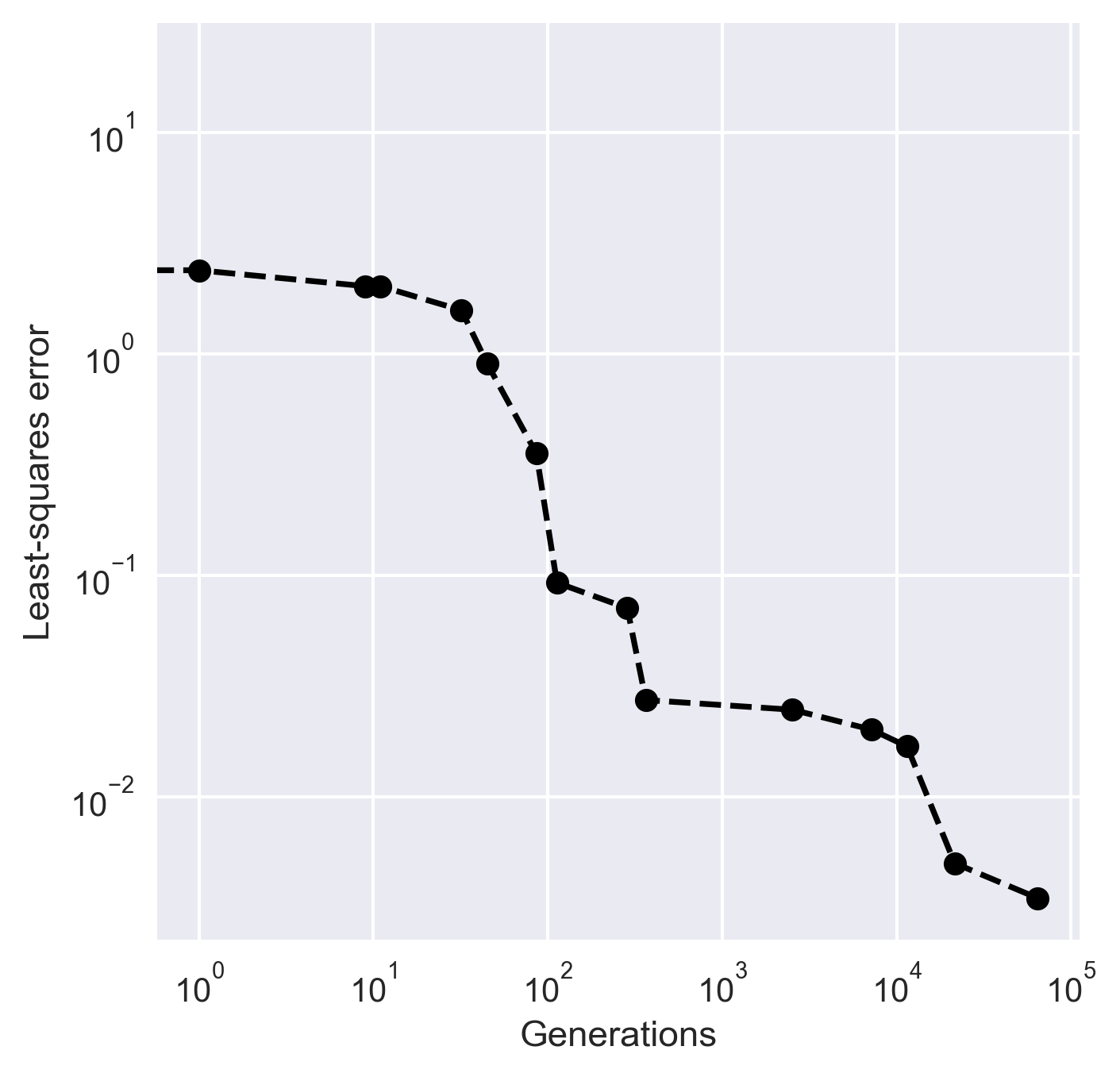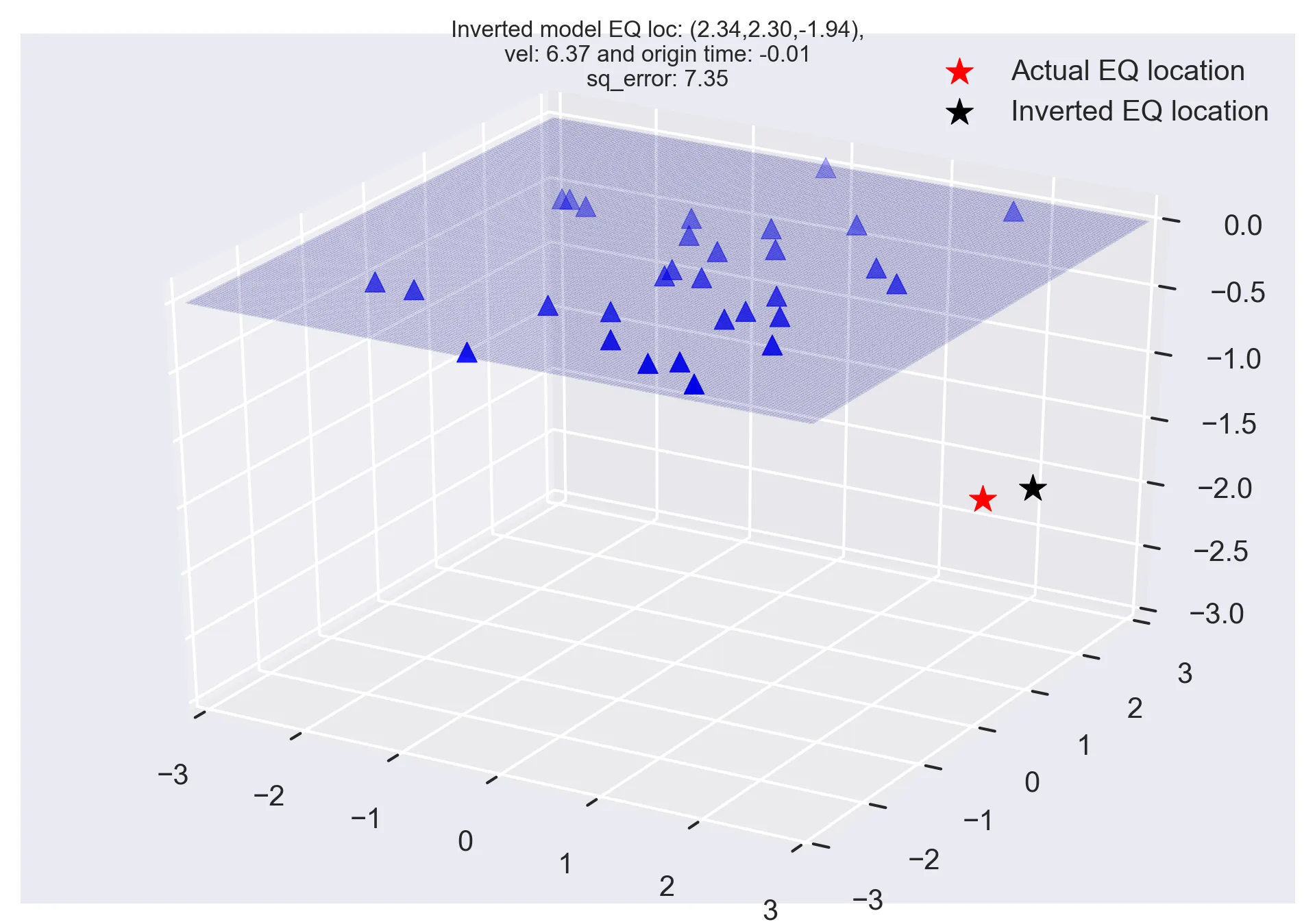# Monte Carlo methods and earthquake location problem (codes included)

The common geophysical problems most often have multimodal objective function with many possible minima. In this post, we will look into the Monte Carlo methods to solve such a hypothetical earthquake location problem.

## Introduction

In the last post, we looked into the introduction of the least-squares approach in geophysical inversion and we formulated a hypothetical earthquake location problem. We saw that we can simply invert for the solution: $\Delta m = (G^T G)^{-1} G^T \Delta d$. This is the simplest approach possible.

## Least-Squares Method in Geosciences

Least-squares method is a popular approach in geophysical inversion to estimate the parameters of a postulated Earth model from given observations. This meth...

The common geophysical problems most often have multimodal objective function with many possible minima (solutions in simple words). Numerical algorithms such as steepest descent, Newton’s method, Quasi-Newton method, conjugate gradient method etc. (Kutz, 2013) tends to get trapped in the local minima if the initial parameters are not wisely chosen. Because of this reason, we seek some global search methods. In this post, we will look into the Monte Carlo methods to solve such problems.

## Similar posts

#### How to plot the boundaries of the states on the basemap of the usaFigure 1: Hypothetical objective functions with global and local minima.

## Monte Carlo Methods

Monte Carlo methods are widely used heuristic optimization technique. It makes clever sample from the parameter space to simulate the working of the complex system and aims to quickly converge for the optimal solution of the objective function. There are several flavors of Monte Carlo methods employed for different applications. The essence of Monte Carlo methods is the search for better sets of model parameters by iteratively generating random set of model parameters in each generation and computing for the least- squares error in the predicted and observed arrival times. The solution with the lowest least- squares error is the accepted solution.

Let us try to solve the earthquake location problem introduced in the previous post using the Monte Carlo method. The method is controlled mainly on two termination criteria - the maximum number of generations (or iterations) of the computation and the difference of the squared error obtained from the current set of model parameters and the squared error obtained from the previous best solution (tolerance value). If the least- squares errors in the predicted and observed arrival times at all the stations is less than the predefined tolerance value or the current generation number is equal to the predefined the number the maximum generations, the results are presented.

The least-squares error for this problem can be written as:

\begin{equation} \label{eq:square} \begin{split} lse = \sum_{i=1}^{N} (d_i^{obs} - d_i^{pre})^2 \end{split} \end{equation}

where N is the number of stations, $d_i^{obs}$ and $d_i^{pre}$ are the observed and predicted arrival times at each station.

The steps involved in Monte Carlo methods are:

1. Define the termination criteria values: maximum number of iterations, and/or tolerance value.
2. Define the lower and upper limits of the model parameters.
3. Set the initial least-squares error value ($lse$). It is best to take it fairly large.
4. Randomly generate the model parameters (earthquake coordinates, velocity and origin time) within the predefined limits (see Table 1)
5. Compute the predicted arrival times at each station using the generated model parameters in step 4.
6. Compute the $lse$ using the predicted arrival times for the current generation and the observed arrival times at each station
7. If the error in the current generation is lower than the previous lowest $lse$, the best model parameters are updated using the current value.
8. Steps 4-7 is repeated until any one of the termination criteria is satisfied.
9. Once the termination criteria are satisfied, the best model parameters are presented.

Table 1: Lower and upper limits of the model parameters for the Monte Carlo solution to earthquake location problem

Model Parameter Min Value Max Value
Earthquake x-coordinate -3 3
Earthquake y-coordinate -3 3
Earthquake z-coordinate -3 0
Velocity 5 7
Origin Time -1 1

I made the maximum generations to be fairly large (100,000) and the tolerance value as $10^3$. The model parameters value at each generation are generated randomly within the predefined range (see Table 1). The obtained best model parameters solution is [2.34, 2.30, -1.94, 6.37, -0.01].

### Import libraries

import matplotlib.pyplot as plt
import numpy as np
import pandas as pd
from mpl_toolkits.mplot3d import Axes3D
from matplotlib import cm

np.random.seed(0)

plt.style.use('seaborn')


### Set up stations to record earthquake

minx, maxx = -2, 2
miny, maxy = -3, 3
numstations = 30
stn_locs=[]
xvals = minx+(maxx-minx)*np.random.rand(numstations)
yvals = miny+(maxy-miny)*np.random.rand(numstations)

for num in range(numstations):
stn_locs.append([xvals[num],yvals[num],0])


### Set up the earthquake

eq_loc = [2,2,-2]
vel = 6 #kmps
origintime = 0

def calc_arrival_time(eq_loc, stnx, stny, stnz, vel, origintime):
eqx, eqy, eqz = eq_loc
dist = np.sqrt((eqx - stnx)**2 + (eqy - stny)**2 + (eqz - stnz)**2)
arr = dist/vel + origintime
return arr


### Make the earthquake arrivals noisy

d_obs = []
noise_level_data = 0.001
for stnx, stny, stnz in stn_locs:
arr = calc_arrival_time(eq_loc, stnx, stny, stnz, vel, origintime)
sign = np.random.choice([-1,1])
d_obs.append(arr+sign*noise_level_data*arr)

d_obs = np.array(d_obs)


### Monte Carlo Solution

def get_rand_number(min_value, max_value):
range_vals = max_value - min_value
choice = np.random.uniform(0,1)
return min_value + range_vals*choice

## Monte Carlo

num_iterations = 100000
inv_model = []
squared_error0 = 100000

mineqx, maxeqx = -3, 3
mineqy, maxeqy = -3, 3
mineqz, maxeqz = 0, -3
gen_num = []
lse = []
for i in range(num_iterations):
eqx0 = get_rand_number(mineqx, maxeqx)
eqy0 = get_rand_number(mineqy, maxeqy)
eqz0 = get_rand_number(mineqz, maxeqz)
vel0 = get_rand_number(5, 7)
origintime0 = get_rand_number(-1, 1)
d_pre = []
for stnx, stny, stnz in stn_locs:
d_pre.append(calc_arrival_time([eqx0, eqy0, eqz0], stnx, stny, stnz, vel0, origintime0))
d_pre = np.array(d_pre)

squared_error = np.sum((d_obs-d_pre)**2)
if squared_error < squared_error0:
print(i,squared_error)
gen_num.append(i)
lse.append(squared_error)
m0 =  np.array([eqx0, eqy0, eqz0, vel0, origintime0])
if np.abs(squared_error-squared_error0)<0.001:
print("Terminated based on tol. value",np.abs(squared_error-squared_error0))
break
squared_error0 = squared_error
inv_model = m0

print("{:.2f} {:.2f} {:.2f} {:.2f} {:.2f}".format(inv_model,inv_model,inv_model,inv_model,inv_model))


### Plot least squares error corresponding to each generations

fig, ax = plt.subplots(1,1,figsize=(5,5))
ax.loglog(gen_num,lse, 'ko--')
ax.set_xlabel('Generations')
ax.set_ylabel('Least-squares error')
plt.savefig('iterations.png',bbox_inches='tight',dpi=300)
plt.close('all')Figure 2:The convergence of least-squares error to find the best model parameters

### Plot the solution

## to create the surface
X = np.linspace(-3, 3, 200)
Y = np.linspace(-3, 3, 200)
X, Y = np.meshgrid(X, Y)
Z = (X**2 + Y**2)*0

fig = plt.figure()
ax = plt.axes(projection='3d')
# plot stations
ax.scatter([x for x in stn_locs],[x for x in stn_locs],[x for x in stn_locs],c='b',marker='^',s=50)
# plot surface
surf = ax.plot_surface(X, Y, Z, rstride=1, cstride=1, cmap=cm.jet,linewidth=0, antialiased=False,alpha=0.1)
# plot actual EQ
ax.scatter(eq_loc,eq_loc,eq_loc,c='r',marker='*',s=100,label='Actual EQ location')
ax.scatter(inv_model,inv_model,inv_model,c='k',marker='*',s=100,label='Inverted EQ location')
plt.title("Inverted model EQ loc: ({:.2f},{:.2f},{:.2f}),\nvel: {:.2f} and origin time: {:.2f}\nsq_error: {:.2f}".format(inv_model,inv_model,inv_model,inv_model,inv_model,squared_error),fontsize=8)
ax.set_xlim([-3,3])
ax.set_ylim([-3,3])
ax.set_zlim(-3,0.1)
plt.legend()

plt.savefig('Earthquake_loc_monte_carlo.png',bbox_inches='tight',dpi=300)
plt.close('all')Figure 3: Hypothetical earthquake location solution using Monte Carlo method.
The actual earthquake location is (2,2,-2), velocity of the homogeneous medium is 6 unit/s, and the actual origin time is 0s. The location of the 30 stations and the actual and inverted earthquake location.

## Genetic Algorithm: a highly robust inversion scheme for geophysical applications

An introduction to the basics of genetic algorithm along with a simple numerical example and solution of an earthquake location problem

Tags:

Categories:

Created on:

#### Disclaimer of liability

The information provided by the Earth Inversion is made available for educational purposes only.

Whilst we endeavor to keep the information up-to-date and correct. Earth Inversion makes no representations or warranties of any kind, express or implied about the completeness, accuracy, reliability, suitability or availability with respect to the website or the information, products, services or related graphics content on the website for any purpose.

UNDER NO CIRCUMSTANCE SHALL WE HAVE ANY LIABILITY TO YOU FOR ANY LOSS OR DAMAGE OF ANY KIND INCURRED AS A RESULT OF THE USE OF THE SITE OR RELIANCE ON ANY INFORMATION PROVIDED ON THE SITE. ANY RELIANCE YOU PLACED ON SUCH MATERIAL IS THEREFORE STRICTLY AT YOUR OWN RISK.